# Gluing lemma for closed subsets

## Statement

Let$A$ and$B$ be closed subsets of a topological space$X$, and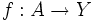$f:A \to Y$ and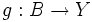$g:B \to Y$ be continuous maps such that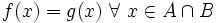$f(x) = g(x) \ \forall \ x \in A \cap B$. Then there exists a unique continuous map from$A \cup B$ to$Y$ whose restriction to$A$ is$f$ and to$B$ is$g$.

The result can be modified to handle finitely many closed sets which cover$X$; however, it does not cater to arbitrarily many closed sets. This is in contrast with the gluing lemma for open subsets.

## Proof

The proof uses the following key facts:

• A map is continuous if and only if the inverse image of any closed subset is closed
• A closed subset of a closed subset is closed. For full proof, refer: Closedness is transitive
• A union of two closed subsets is closed

### Proof details

Given: A topological space$X$, closed subsets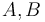$A,B$ of$X$. Continuous functions$f,g:A \cup B \to Y$, such that$f|_{A \cap B} = g|_{A \cap B}$.

To prove: There is a unique continuous map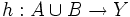$h:A \cup B \to Y$ whose restriction to$A$ equals$f$ and whose restriction to$B$ equals$g$.

Proof: Note that since$A,B$ is closed in$X$, they are also closed in$A \cup B$.

1. There is a unique function$h$ on$A \cup B \to Y$ whose restriction to$A$ is$f$ and to$B$ is$g$: This is set-theoretically obvious.
2. This function is continuous: For this, we prove that the inverse image of any closed subset of$Y$ is closed in$A \cup B$. Let$C$ be a closed subset of$Y$.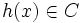$h(x) \in C$ iff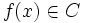$f(x) \in C$ or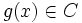$g(x) \in C$. Thus,$h^{-1}(C) = f^{-1}(C) \cup g^{-1}(C)$. Since$f$ is continuous,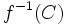$f^{-1}(C)$ is a closed subset of$A$, which is closed in$A \cup B$. So$f^{-1}(C)$ is closed in$A \cup B$. Similarly,$g^{-1}(C)$ is closed in$A \cup B$. Since a finite union of closed subsets is closed,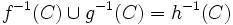$f^{-1}(C) \cup g^{-1}(C) = h^{-1}(C)$ is closed in$A \cup B$.

## Applications

The gluing lemma for closed subsets is one of the many results in point-set topology which is applied everywhere, often without even consciously realizing it. Here are some examples:

• The multiplication defined in the fundamental group and higher homotopy grooups, uses the gluing lemma (to argue that a composite of loops is a loop)
• The fact that homotopies can be composed also uses the gluing lemma
• Many of the proofs involving manifolds, for instance, the proof that the inclusion of a point in a manifold is a cofibration, or the proof that connected manifolds are homogeneous, uses the gluing lemma; we glue an explicit map in a neighbourhood of the point with a constant map outside.